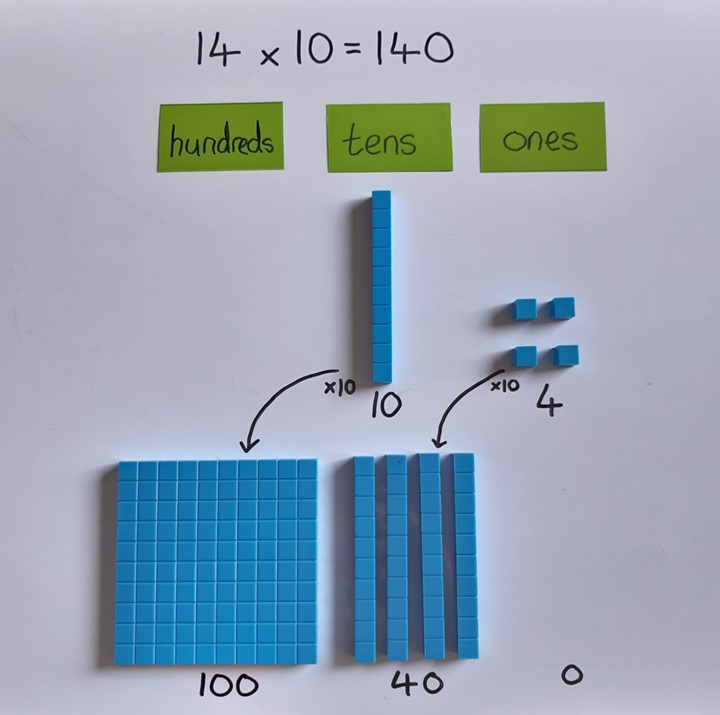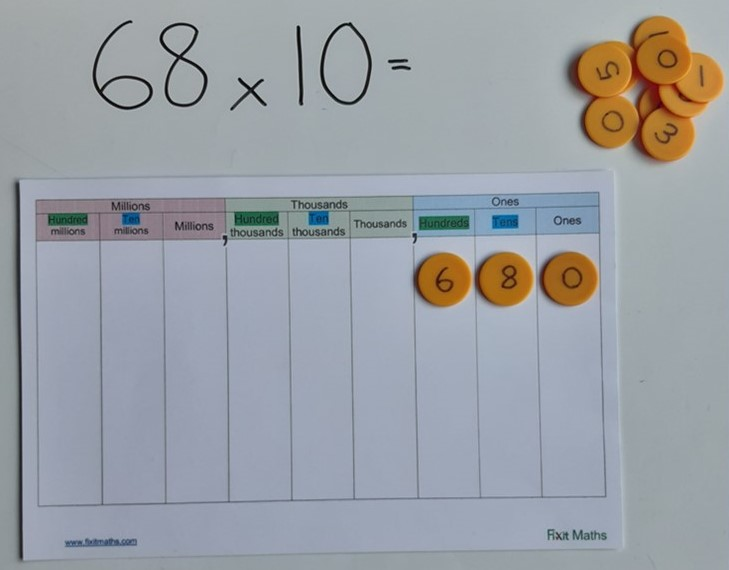## Multiplying by 10

When a number is multiplied by 10, each part of that number becomes 10 times larger. So a one becomes a ten, a ten becomes a hundred etc. This can easily be seen using Dienes, where 10 one cubes can be put together to make a ten stick, and 10 ten sticks can be put together to make a hundred flat.Using a place value grid, we can see that the digits move one place to the left as they are multiplied by 10, representing how the value of each has become 10 times larger. A place holder is needed to show that there are now no ones.Download an activity pack of games to reinforce multiplying or dividing by 10, 100 or 1000
Click here to generate worksheet to practise multiplying and dividing by 10, 100 & 1000.